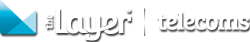### Knowledgebase

### Price books: Understanding 'OGR Buy Price Override'

OGR buy price override field is required for tariffs, which provide you with recurring margin as well as OGR (ongoing revenue)

In the examples below we will show you how profits are calculated when OGR buy price override is calculated as TRUE and FALSE

## OGR Buy Price Override = TRUE

When OGR buy price override is marked as TRUE the profits are calculated as follows:

Profit = Recurring Margin + OGR

Recurring Margin = (Recurring Sell – Recurring Buy) x Term

OGR = Recurring Buy x Term x OGR

### Example:

Tariff: O2 Sharer

Recurring Sell: £15.48

Term: 24 months

OGR: 35%

OGR Term: 24Profit for one service:

Recurring margin: (£15.48 - £10) x 24 = £131.52

OGR: £10 x 24 x 35% = £84

Profit: £131.52 + £84 = £215.52## OGR Buy Price Override = FALSE

When OGR buy price override is marked as FALSE the profits are calculated as follows:

Profit = Recurring Margin + OGR

Recurring Margin = (Recurring Sell – Recurring Buy) x Term

OGR = Recurring Sell x Term x OGR

### Example:

Tariff: O2 Sharer

Recurring Sell: £15.48

Term: 24 months

OGR: 35%

OGR Term: 24 monthsProfit for one service:

Recurring margin: (£15.48 - £10) x 24 = £131.52

OGR: £15.48 x 24 x 35% = £130.03

Profit: £131.52 + £130.03 = £261.55Author: Michaela Gormanova